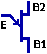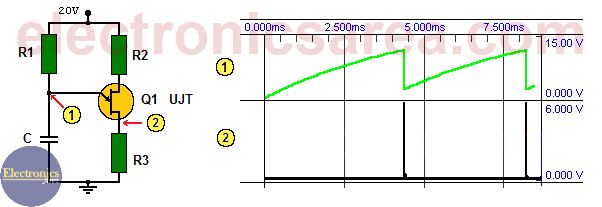# UJT Relaxation oscillator circuit

The UJT relaxation oscillator circuit is a non linear oscillator used to generate a nonsinusoidal repetitive output signals that can trigger power control devices such as SCRs and TRIACs. The UJT acronim comes from: Unijunction Transistor.

## How the relaxation oscillator using UJT transistor work?

The capacitor C is charged until it reaches the UJT trigger voltage. When this happens, it discharges through the UJT E-B1 junction. (See the UJT diagram).UJT Symbol (Unijunction Transistor)

The capacitor is discharged until its voltage reaches what is called the “Valley voltage” (Vv) which is approximately 2.5 volts.  Al that moment the UJT transistor turns off (there is no current conduction through the E-B1 junction) and the capacitor starts charging again. (See the green line on the diagram below).The black line (right – bottom on diagram) shows the voltage across the resistor R3 when the capacitor discharges

If we want to change the oscillation frequency, we can change the capacitor value, the R1 resistor value or both. Resistors R2 and R3 are also important to find the frequency of oscillation.## UJT Relaxation oscillator frequency formula

The oscillation frequency is approximately given by: F = 1/R1C. It is very important to know that resistor R1 must have values that should be in an acceptable range for the circuit to oscillate.

These values are obtained using the following formulas: R1 max = (Vs-Vp)/Ip, R1 min = (Vs-Vv)/Iv. where:

• Vs = Power supply voltage value (20 volts on this circuit)
• Vp = value obtained depending on the particular UJT parameters
• Ip = manufacturer’s specification
• Vv = manufacturer’s specification
• Iv = manufacturer’s specification

## List of components for the UJT Relaxation oscillator circuit

• 1  2N4870, 2N2646 or similar unijunction transistor (UJT)
• 1  50 KΩ resistor (R1)
• 1  330 Ω resistor (R2)
• 1  47 Ω resistor (R3)
• 1  0.1 uF capacitor (C)
• 0ther: 20 volts power supply (a 12 or 9 volt battery will work fine).

• 2
•
•
•
•
•
•
•
•
2
Shares
•
2
Shares
• 2
•
•
•
•
•
•
•